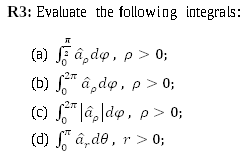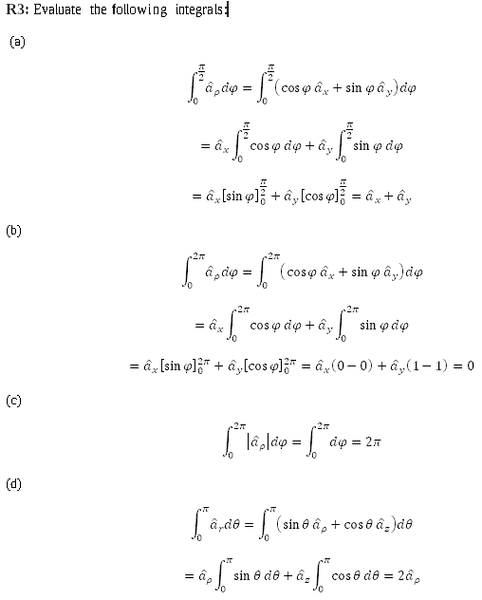# Taking the integral of vectors?

• I
• Vishera
In summary, the conversation discusses the use of different coordinate systems and their corresponding unit vectors in vector integrals. The basis vector for cylindrical coordinates, ##\hat{a}_{\rho}##, depends on the angle ##\phi## while the basis vector for rectangular coordinates, ##\hat{a}_{x}##, does not. This is due to the fact that the basis vector is defined by the coordinate system, and the coordinates themselves can vary depending on the point in space. The conversation also touches on the need for a good resource to understand vector integrals and clarifies the meaning of the unit vector, ##\hat{a}_{\rho}##.

#### Vishera

Question:Solution:The notation used is: ##(x,y,z)## is for rectangular coordinates, ##(\rho,\varphi,z)## for cylindrical coordinates and ##(r,\theta,\varphi)## for spherical coordinates. ##{ { \hat { a } } }_{ ρ }## represents the unit vector for ##\rho## (same applies to ##x, y, z## and other coordinates).

In part a, can't you take out ##{ { \hat { a } } }_{ ρ }## from the integral? I'm having trouble understanding how ##{ { \hat { a } } }_{ ρ }## depends on ϕ. ##{ { \hat { a } } }_{ ρ }## is defined as ρ=1, ϕ=0 and z=0. Aren't all of these constants that do not rely on ϕ?

•dsaun777
Vishera said:
n part a, can't you take out ^aρa^ρ{ { \hat { a } } }_{ ρ } from the integral? I'm having trouble understanding how ^aρa^ρ{ { \hat { a } } }_{ ρ } depends on ϕ. ^aρa^ρ{ { \hat { a } } }_{ ρ } is defined as ρ=1, ϕ=0 and z=0. Aren't all of these constants that do not rely on ϕ?
No, definitely not. The components might be constant, but the basis vector itself depends on the angle! Just draw the basis vector at a few points on a piece of paper and you will see that it does.

Orodruin said:
No, definitely not. The components might be constant, but the basis vector itself depends on the angle! Just draw the basis vector at a few points on a piece of paper and you will see that it does.

Sorry, I'm having trouble understanding. I'm a little rusty on linear algebra. The basis is "vectors that are linearly independent and every vector in the vector space is a linear combination of this set". But you can only take the basis of a vector space. Are you saying I should take the basis of the cylindrical coordinates space?

Do you have any good resource where I can read more about integrals of vectors?

If you use curvilinear coordinates, the basis used depends on the point in space.

It seems this is going over my head. Do you know what I can read to understand vector integrals?

I already completely Calculus 3, and I did double integrals, triple integrals, line integrals, surface integrals, green's theorem, stoke's theorem, divergence theorem, etc. But I don't recall ever doing vector integrals and now we're expected to do vector integrals but the class/textbook never covered it.

It seems to me that your problem is in understanding that the vector basis depends on the position, not vector integrals. Vector integrals work just the same as other integrals, but you cannot move things which are not constant out if the integral.

Alright, thank you for mentioning that vector integrals work like scalar integrals.

You said earlier that "If you use curvilinear coordinates, the basis used depends on the point in space." I'm not sure what you're referring to when you say "the point in space" since the integral in the question doesn't seem to give any point in space. I can see from the limits of integration, we're suppose to integrate from ϕ=0 to ϕ=pi/2. Are you suggesting that we're actually integrating from ##(\rho=1,\varphi=0,z=0)## to ##(\rho=1,\varphi=\pi/2,z=0)##?

Vishera said:
Alright, thank you for mentioning that vector integrals work like scalar integrals.

You said earlier that "If you use curvilinear coordinates, the basis used depends on the point in space." I'm not sure what you're referring to when you say "the point in space" since the integral in the question doesn't seem to give any point in space. I can see from the limits of integration, we're suppose to integrate from ϕ=0 to ϕ=pi/2. Are you suggesting that we're actually integrating from ##(\rho=1,\varphi=0,z=0)## to ##(\rho=1,\varphi=\pi/2,z=0)##?
Yes, although not necessarily those values of rho and z, the problem does not specify this, but the basis vectors only depend on phi.

So to simplify things, would I be correct in saying that ##{ { \hat { a } } }_{ ρ }## depends on ##\varphi## because the cylindrical coordinate system has ##\varphi## as one of its components but ##{ { \hat { a } } }_{ x }## does not depend on ##\varphi## because the rectangular coordinate system does not have ##\varphi## as one of its components?

Thanks for the help.

For anyone reading this in the future: I've figured it out.

The unit vector ##\hat{a}_{\rho}## does not mean: ##\rho=1##, ##\phi=0##, ##z=0##. ##\hat{a}_{\rho}## actually depends on ##\phi## as it's ##\left( cos\phi,sin\phi,0 \right)##.